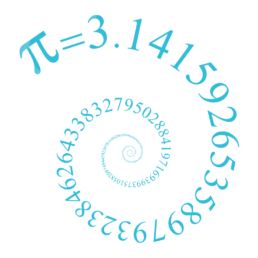Bournemouth Maths Tutor Barney M-T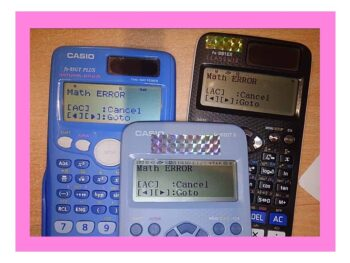What does “MATH ERROR” mean?

SHORT ANSWER: this means you have just asked the calculator to do a sum it doesn’t like. The three most common reasons for getting this error message are: 1) you have tried to divide by zero: forbidden in maths! If you type in $3\div 0$ you are asking the calculator “zero times by what equalsWHAT’S ZERO TIMES BY INFINITY?

SHORT ANSWER: 0 x ∞ = anything you like! Intrigued? Read on… LONG ANSWER: the question seems absurd: after all, zero multiplied by anything is zero, yet any multiple of infinity is always infinity. But 0 x ∞ cannot be both zero and infinity, can it? Infinity doesn’t behave in the same way as other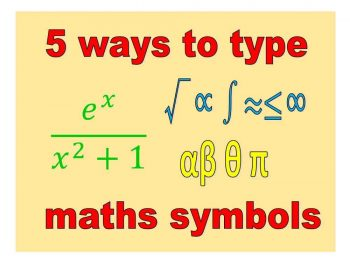5 WAYS TO TYPE SYMBOLS π λ √∫≤∑≈

not to mention $\frac{e^{-t}}{y^3+1}$ To type Mathematical Symbols on your PC: 1) USE WINDOWS EMOJIS: To type ⅷ∀√∛∞∑²³ⁿ∃∞∫≈≡≥ and many more. Simply press windows key together with .  to bring up the emoji keyboard, then select Ω or ∞to bring up the maths palette. VERDICT: works with any app on PC including Facebook, Twitter, MSHere I am at the World’s Steepest Road in Harlech, North Wales. The road sign indicates an almighty 40% – but how is this measured? ON ROADS: The 40% means that however far you travel horizontally, you travel 40% of that vertically. So if you go 100m across, you go 40m up. SO A ROADUSING MATHS TO CHECK WORLD RECORD ATTEMPTS: PETE’S DIABOLO HIGH THROW

My friend Pete can throw a diabolo ridiculously high – check out this video of him in action! Pete thinks his throw is higher than the “official” world record of 23.92metres so asked me if maths could measure his throw. So, how about it, maths? METHOD 1 (not recommended!): get a long tape measure and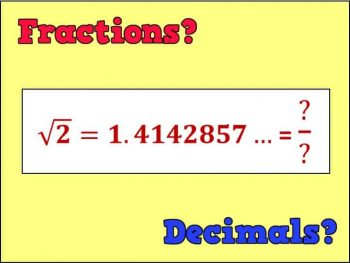WHICH ARE BETTER: FRACTIONS OR DECIMALS?

DECIMALS ARE WAAAY BETTER THAN FRACTIONS: most GCSE students prefer decimals because they allow you to compare the sizes of two numbers at a glance! For instance, which is bigger out of $\frac{2}{5}$ and $\frac{3}{7}$? Um…? But in decimal form we can easily see that $\frac{3}{7}=0.428571…>0.4=\frac{2}{5}$. FRACTIONS RULE SUPREME: fractions allow for easy multiplication, and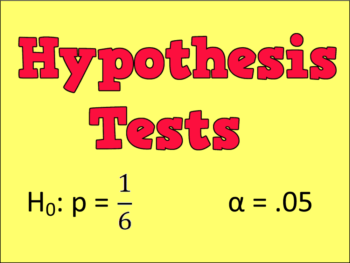HYPOTHESIS TESTS

In statistics, these wonderful techniques allow us to use some data that we have collected to make predictions and conclusions about the real world. AN EXAMPLE: Amy and Bill are playing snakes and ladders, but Amy thinks Bill is cheating because he keeps rolling a six. Bill insists he is just being lucky. They decide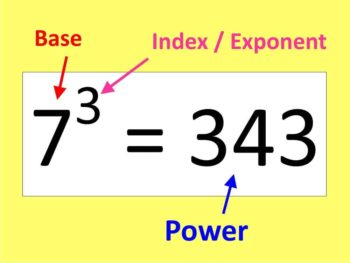WORD OF THE WEEK: INDEX

INDEX (plural indices): you can see these in expressions like $5^2 = 25$. The 5 is called the BASE, the 2 is called the EXPONENT or INDEX, and the 25 is called a power (in this case it’s a power of 5).